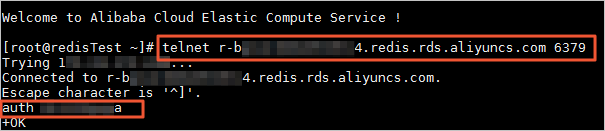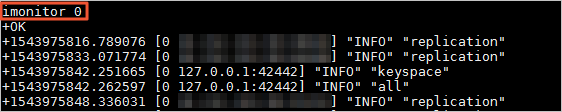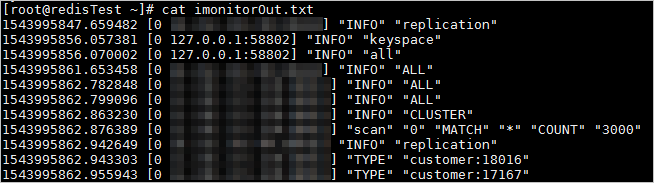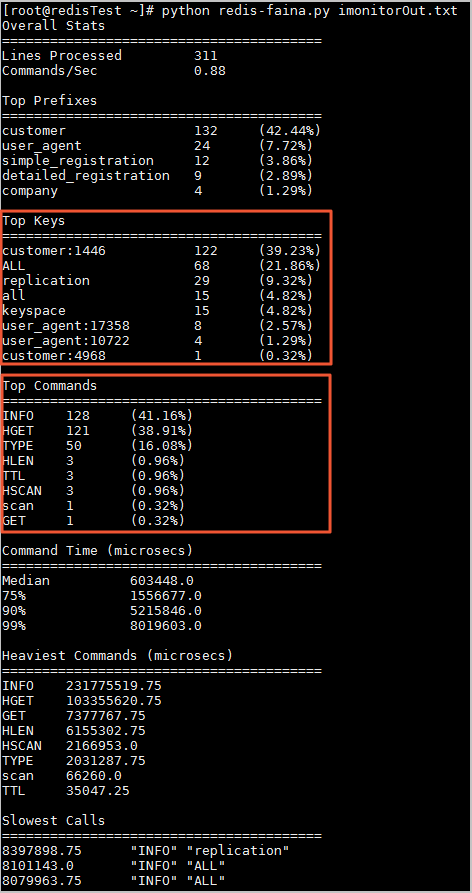You can use the imonitor command developed by Alibaba Cloud to monitor the request status of a specific node in the Redis cluster, and use redis-faina to discover hotkeys and commands from the monitoring data.

## Background information

When you use the ApsaraDB for Redis cluster edition, if the hotkey traffic on a specific node is too large, other services in the server may fail to continue. If the cache of the hotkey exceeds the current cache capacity, the sharding service of the cache will crash.

You can use Performance monitoring and Alert settings to monitor the cluster status in real time and set alert rules. When you discover an overloaded sub-node, you can use the imonitor command to view the client request of the node, and use redis-faina to analyze the hotkey.

## Prerequisites

• You have activated an ECS instance that can interconnect with the ApsaraDB for Redis cluster edition.
• You have installed Python and Telnet in the ECS instance.
Note The sample environment in this topic is CentOS 7.4 and Python 2.7.5.

## Procedure

1. In the ECS instance, use Telnet to connect to the Redis cluster.
1. Use `# telnet <host> <port>` to connect to the Redis cluster.
Note `host` is the connection address of the Redis cluster. `port` is the connection port (the default port number is 6379).
2. Enter `auth <password>` for verification.
Note `password` is the password for the Redis cluster.Note If `+OK` is returned, the connection is successful.
2. Use `imonitor <db_idx>` to collect the request data of the target node.Note

The imonitor command is similar to the info command and the iscan command. This command added a parameter to the monitor command, and the user can specify the node to run the monitor command. In this command, the value range of db_idx is [0, nodecount). You can obtain the value of nodecount by running the info command or viewing the instance topology in the console.

In this example, the value of db_idx of the target node is 0.

If `+OK` is returned, the output of of monitored request records continues.

3. Collect the monitoring data based on your business requirements and enter the QUIT command. Press Enter to close the Telnet connection.
4. Store the monitoring data to a .txt file, and delete the plus sign (+) at the beginning of the line. You can replace this sign by using the text editing tool. The stored file is as follows:5. Create a Python script for request analysis, and save it as redis-faina.py. The code is as follows:
``````#! /usr/bin/env python
import argparse
import sys
from collections import defaultdict
import re

line_re_24 = re.compile(r"""
^(? P<timestamp>[\d\.]+)\s(\(db\s(? P<db>\d+)\)\s)?"(? P<command>\w+)"(\s"(? P<key>[^(? <! \\)"]+)(? <! \\)")?( \s(? P<args>. +))? \$
""", re.VERBOSE)

line_re_26 = re.compile(r"""
^(? P<timestamp>[\d\.]+)\s\[(? P<db>\d+)\s\d+\.\d+\.\d+\.\d+:\d+]\s"(? P<command>\w+)"(\s"(? P<key>[^(? <! \\)"]+)(? <! \\)")?( \s(? P<args>. +))? \$
""", re.VERBOSE)

class StatCounter(object):

def __init__(self, prefix_delim=':', redis_version=2.6):
self.line_count = 0
self.skipped_lines = 0
self.commands = defaultdict(int)
self.keys = defaultdict(int)
self.prefixes = defaultdict(int)
self.times = []
self._cached_sorts = {}
self.start_ts = None
self.last_ts = None
self.last_entry = None
self.prefix_delim = prefix_delim
self.redis_version = redis_version
self.line_re = line_re_24 if self.redis_version < 2.5 else line_re_26

def _record_duration(self, entry):
ts = float(entry['timestamp']) * 1000 * 1000 # microseconds
if not self.start_ts:
self.start_ts = ts
self.last_ts = ts
duration = ts - self.last_ts
if self.redis_version < 2.5:
cur_entry = entry
else:
cur_entry = self.last_entry
self.last_entry = entry
if duration and cur_entry:
self.times.append((duration, cur_entry))
self.last_ts = ts

def _record_command(self, entry):
self.commands[entry['command']] += 1

def _record_key(self, key):
self.keys[key] += 1
parts = key.split(self.prefix_delim)
if len(parts) > 1:
self.prefixes[parts] += 1

@staticmethod
def _reformat_entry(entry):
max_args_to_show = 5
output = '"%(command)s"' % entry
if entry['key']:
output += ' "%(key)s"' % entry
if entry['args']:
arg_parts = entry['args'].split(' ')
ellipses = ' ...' if len(arg_parts) > max_args_to_show else ''
output += ' %s%s' % (' '.join(arg_parts[0:max_args_to_show]), ellipses)
return output

def _get_or_sort_list(self, ls):
key = id(ls)
if not key in self._cached_sorts:
sorted_items = sorted(ls)
self._cached_sorts[key] = sorted_items
return self._cached_sorts[key]

def _time_stats(self, times):
sorted_times = self._get_or_sort_list(times)
num_times = len(sorted_times)
percent_50 = sorted_times[int(num_times / 2)]
percent_75 = sorted_times[int(num_times * . 75)]
percent_90 = sorted_times[int(num_times * . 90)]
percent_99 = sorted_times[int(num_times * . 99)]
return (("Median", percent_50),
("75%", percent_75),
("90%", percent_90),
("99%", percent_99))

def _heaviest_commands(self, times):
times_by_command = defaultdict(int)
for time, entry in times:
times_by_command[entry['command']] += time
return self._top_n(times_by_command)

def _slowest_commands(self, times, n=8):
sorted_times = self._get_or_sort_list(times)
slowest_commands = reversed(sorted_times[-n:])
printable_commands = [(str(time), self._reformat_entry(entry)) \
for time, entry in slowest_commands]
return printable_commands

def _general_stats(self):
total_time = (self.last_ts - self.start_ts) / (1000*1000)
return (
("Lines Processed", self.line_count),
("Commands/Sec", '%. 2f' % (self.line_count / total_time))
)

def process_entry(self, entry):
self._record_duration(entry)
self._record_command(entry)
if entry['key']:
self._record_key(entry['key'])

def _top_n(self, stat, n=8):
sorted_items = sorted(stat.iteritems(), key = lambda x: x, reverse = True)
return sorted_items[:n]

def _pretty_print(self, result, title, percentages=False):
print title
print '=' * 40
if not result:
print 'n/a\n'
return

max_key_len = max((len(x) for x in result))
max_val_len = max((len(str(x)) for x in result))
for key, val in result:
key_padding = max(max_key_len - len(key), 0) * ' '
if percentages:
val_padding = max(max_val_len - len(str(val)), 0) * ' '
val = '%s%s\t(%. 2f%%)' % (val, val_padding, (float(val) / self.line_count) * 100)
print

def print_stats(self):
self._pretty_print(self._general_stats(), 'Overall Stats')
self._pretty_print(self._top_n(self.prefixes), 'Top Prefixes', percentages = True)
self._pretty_print(self._top_n(self.keys), 'Top Keys', percentages = True)
self._pretty_print(self._top_n(self.commands), 'Top Commands', percentages = True)
self._pretty_print(self._time_stats(self.times), 'Command Time (microsecs)')
self._pretty_print(self._heaviest_commands(self.times), 'Heaviest Commands (microsecs)')
self._pretty_print(self._slowest_commands(self.times), 'Slowest Calls')

def process_input(self, input):
for line in input:
self.line_count += 1
line = line.strip()
match = self.line_re.match(line)
if not match:
if line ! = "OK":
self.skipped_lines += 1
continue
self.process_entry(match.groupdict())

if __name__ == '__main__':
parser = argparse.ArgumentParser()
'input',
type = argparse.FileType('r'),
default = sys.stdin,
nargs = '?',
help = "File to parse; will read from stdin otherwise")
'--prefix-delimiter',
type = str,
default = ':',
help = "String to split on for delimiting prefix and rest of key",
required = False)
6. Run the `python redis-faina imonitorOut.txt` command to parse the monitoring data. imonitorOut.txt is the monitoring data stored in the example.